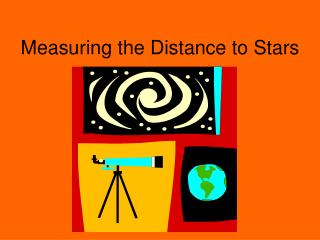DownloadDownload PresentationMeasuring the Distance to Stars

# Measuring the Distance to Stars

Télécharger la présentation## Measuring the Distance to Stars

- - - - - - - - - - - - - - - - - - - - - - - - - - - E N D - - - - - - - - - - - - - - - - - - - - - - - - - - -
##### Presentation Transcript

1. Measuring the Distance to Stars

2. The Parallax Method • To the naked eye stars are so far away that they do not appear to change positions even though we are rotating (8,000 miles wide) and revolving (186, 000,000 miles). • However with the invention of photography, we had new tool to record stars and look for tiny shifts in their position

3. The Parallax Method • The first time we were able to see a tiny shift in the position of a star was in 1916 during the First World War. • The shifts are less than an arcminute and were only possible because of the improvements of photography and telescopes.

4. The Parallax Method

5. The Parallax Method • We use the orbit of the Earth to make our measurements. • By taking pictures 6 months apart we have moved about 186,000,000 miles. • This change of position makes the nearby stars appear to shift compared to the more distant stars. • By recording the angle of the shift we can calculate the distance to the star.

6. The Parallax Method • For very small angles we can assume that the sine of the angle is very close to the value of the angle itself (using radians as the measure). • Therefore: Sin θ = opp/hyp and • Hyp = opp/sin θ or Hyp = opp/ θ

7. The Parallax Method • However, to simplify things astronomer have developed an easier formula based on the parsec. • 1 parsec = 3.26 light year, where • 1 light year = 5.88 trillion miles • The formula is d = 1/p where d is distance in parsecs and p is the parallax measured in arc seconds.

8. The Parallax Method